# Quiz 20: Inventory Management: Economic Order Quantity, Jit, and the Theory of Constraints

Business

Ordering Cost is the Cost of placing a purchase order as well as receiving the purchase order. Carrying Cost is the Cost of holding the inventory which includes warehousing cost such as rent, salaries etc. Total Cost is the aggregate of Ordering Cost and Carrying Cost. Economic Order Quantity (EOQ) is the quantity which minimizes the Total Holding cost and Carrying Cost. 1.The following information of T ltd is given in question: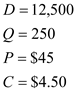Where, D is Annual Demand, Q is Lot size for production, P is cost of placing an order and C is carrying cost per unit.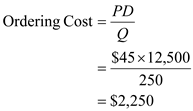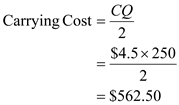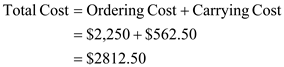2.Calculation of EOQ is done as follows: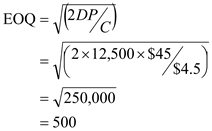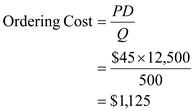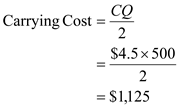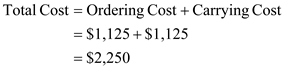Relative to the current order quantity, the size of Economic Order Quantity is larger this results in less number of orders. However, the total cost decreases by \$562.50 (\$2,812.50-\$2,250). Further, Ordering Cost and Carrying Cost are equal by considering Economic order quantity. 3.If ordering cost is reduced to \$0.45 per order, the EOQ will be as follows: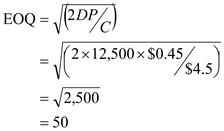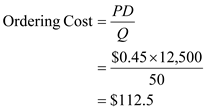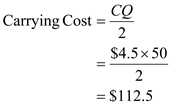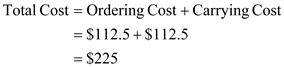The smaller quantity of orders will reduce the cost of inventory. This results in more use just-in-time purchasing of inventory.

Ordering costs: Ordering costs are the costs of placing and receiving an order. For example, insurance for shipment, unloading costs, etc. Setup costs: Setup costs refer to the costs of preparing equipment and facilities thereby producing a particular product or component. For example, wages of idled production workers, costs of test runs, etc. Carrying costs: Carrying costs are the costs of holding inventory. For example, inventory taxes, storage space, etc.

1.Calculation of Reorder point: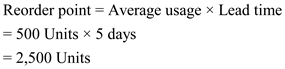Hence, the Reorder point is 2,500 Units ________________________________________________________________________ 2.Graphical representation: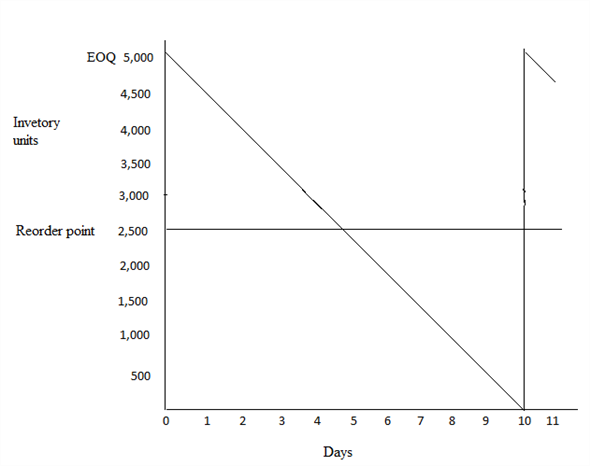Calculation of reorder point when the uncertainty exists: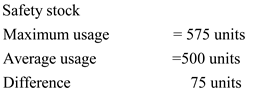The safety stock is calculated using the following formula,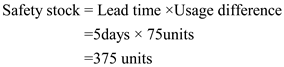Hence, the safety stock is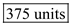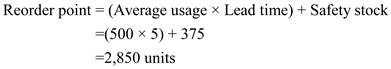Hence, the reorder point is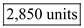There is no answer for this question

There is no answer for this question

There is no answer for this question